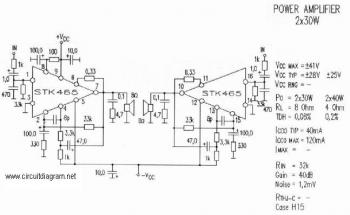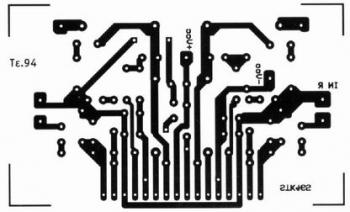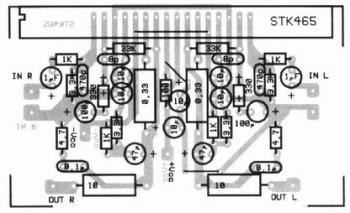# 2 x 30W Audio Amplifier with STK-465

Here is the simple 2 x 30W audio amplifier with STK-465 power IC. Simple circuit with higher power output, this amplifier circuit is very easy to build. Just look at the PCB layout of this circuit.. 🙂 . The active component required is just the main power amplifier chip STK-465.

Schematic diagram:This is a stereo audio system application. A STK-465 amplifier capable to deliver 2 x 30W audio output at 8 ohm loudspeaker and 2 x 40W using 4 ohm loadspeaker.

Components list:

 R1 = 1K C1 = 1uF/35V R2 = 3,3K C2 = 470pF R3 = 100 C3 = 100uF/60V R4 = 330 C4 = 100uF/60V R5 = 3,3K C5 = 10uF/60V R6 = 1K C6 = 47uF/60V R7 = 0,33 C7 = 8,2pF R8 = 33k C8 = 0,1uF R9 = 4,7 C9 = 1uF/35V R10 = 1k C10 = 470pF R11 = 3,3k C11 = 100uF/60V R12 = 100 C12 = 100uF/60V R13 = 330 C13 = 10uF/60V R14 = 3,3k C14 = 47uF/60V R15 = 1k C15 = 8,2pF R16 = 0,33 C16 = 0,1uF R17 = 33k R18 = 4,7 IC1 = STK465 LS1 = Speaker 40W 8 or 4 Ohm

PCB Layout:Component placement:This circuit uses dual polarity power supply to work. Maximum supply voltage is 41V while the recommended supply voltage is 28V.

Sample Scheme of Dual Polarity Power Supply:Download STK-465 datasheet You will need the datasheet for project reference.

Incoming Search: stk465 stereo amplifier, stk465, stk 465, stk465 amp circuit diagrams

This site uses Akismet to reduce spam. Learn how your comment data is processed.